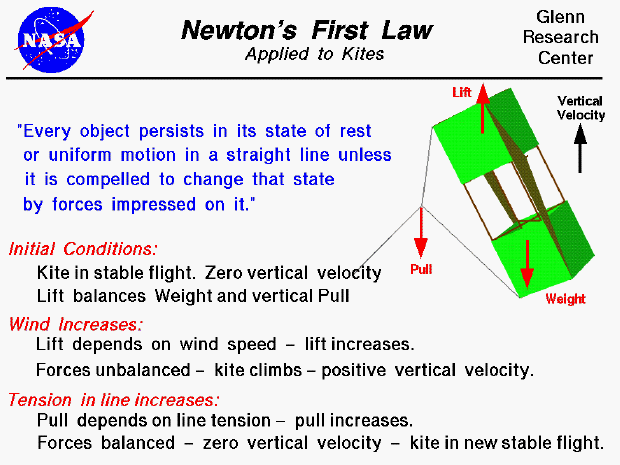Sir Isaac Newton first presented his three laws of motion in the "Principia Mathematica Philosophiae Naturalis" in 1686. His first law states that every object will remain at rest or in uniform motion in a straight line unless compelled to change its state by the action of an external force. This is normally taken as the definition of inertia. The key point here is that if there is no net force resulting from unbalanced forces acting on an object (if all the external forces cancel each other out), then the object will maintain a constant velocity. If that velocity is zero, then the object remains at rest. And if an additional external force is applied, the velocity will change because of the force. The amount of the change in velocity is determined by Newton's second law of motion.

A kite flying through the air is a good example of this principle. The forces acting on a kite in flight include the weight, the aerodynamic lift and drag, and the pull of the control line. In stable flight, these forces are all balanced and the kite holds a fixed altitude. The vertical velocity of the kite is zero. If the wind increases slightly, the lift and drag increase because the aerodynamic forces depend on the square of the velocity. The forces on the kite are no longer balanced and there is a net vertical force on the kite. The kite moves vertically because the lift now exceeds the weight and the vertical pull. As the kite climbs, the tension force increases because of increased drag. The vertical pull depends on the tension force, so the vertical pull increases. Eventually a new balance point is established in which the lift balances the weight and the vertical pull. The kite then achieves a new stable flight condition at a slightly higher altitude. If the wind decreases, the lift, drag, and vertical pull all are decreased and the kite achieves a new stable flight condition at a lower altitude.Guided Tours

Beginner's Guide to Aerodynamics
Beginner's Guide to Propulsion
Beginner's Guide to Model Rockets
Beginner's Guide to Kites
Beginner's Guide to Aeronautics